moveit2 The MoveIt Motion Planning Framework for ROS 2.
moveit::core::JointModel Class Referenceabstract

A joint from the robot. Models the transform that this joint applies in the kinematic chain. A joint consists of multiple variables. In the simplest case, when the joint is a single DOF, there is only one variable and its name is the same as the joint's name. For multi-DOF joints, each variable has a local name (e.g., x, y) but the full variable name as seen from the outside of this class is a concatenation of the "joint name"/"local name" (e.g., a joint named 'base' with local variables 'x' and 'y' will store its full variable names as 'base/x' and 'base/y'). Local names are never used to reference variables directly. More...

`#include <joint_model.h>`

Inheritance diagram for moveit::core::JointModel: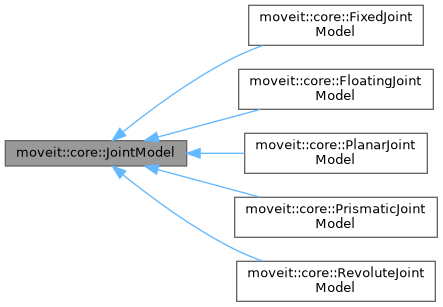[legend]
Collaboration diagram for moveit::core::JointModel: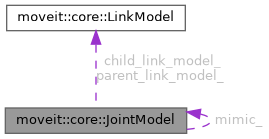[legend]

## Public Types

enum  JointType {
UNKNOWN , REVOLUTE , PRISMATIC , PLANAR ,
FLOATING , FIXED
}
The different types of joints we support. More...

using Bounds = std::vector< VariableBounds >
The datatype for the joint bounds. More...

## Public Member Functions

JointModel (const std::string &name, size_t joint_index, size_t first_variable_index)
Constructs a joint named name. More...

virtual ~JointModel ()

const std::string & getName () const
Get the name of the joint. More...

JointType getType () const
Get the type of joint. More...

std::string getTypeName () const
Get the type of joint as a string. More...

Get the link that this joint connects to. The robot is assumed to start with a joint, so the root joint will return nullptr here. More...

Get the link that this joint connects to. There will always be such a link. More...

virtual double distance (const double *value1, const double *value2) const =0
Compute the distance between two joint states of the same model (represented by the variable values) More...

double getDistanceFactor () const
Get the factor that should be applied to the value returned by distance() when that value is used in compound distances. More...

void setDistanceFactor (double factor)
Set the factor that should be applied to the value returned by distance() when that value is used in compound distances. More...

virtual unsigned int getStateSpaceDimension () const =0
Get the dimension of the state space that corresponds to this joint. More...

const JointModelgetMimic () const
Get the joint this one is mimicking. More...

double getMimicOffset () const
If mimicking a joint, this is the offset added to that joint's value. More...

double getMimicFactor () const
If mimicking a joint, this is the multiplicative factor for that joint's value. More...

void setMimic (const JointModel *mimic, double factor, double offset)
Mark this joint as mimicking mimic using factor and offset. More...

const std::vector< const JointModel * > & getMimicRequests () const
The joint models whose values would be modified if the value of this joint changed. More...

Notify this joint that there is another joint that mimics it. More...

Get all the link models that descend from this joint, in the kinematic tree. More...

const std::vector< const JointModel * > & getDescendantJointModels () const
Get all the joint models that descend from this joint, in the kinematic tree. More...

const std::vector< const JointModel * > & getNonFixedDescendantJointModels () const
Get all the non-fixed joint models that descend from this joint, in the kinematic tree. More...

bool isPassive () const
Check if this joint is passive. More...

void setPassive (bool flag)

virtual void interpolate (const double *from, const double *to, const double t, double *state) const =0
Computes the state that lies at time t in [0, 1] on the segment that connects from state to to state. The memory location of state is not required to be different from the memory of either from or to. More...

virtual double getMaximumExtent (const Bounds &other_bounds) const =0
Get the extent of the state space (the maximum value distance() can ever report) More...

double getMaximumExtent () const

Reason about the variables that make up this joint
const std::vector< std::string > & getVariableNames () const
Get the names of the variables that make up this joint, in the order they appear in corresponding states. For single DOF joints, this will be just the joint name. For multi-DOF joints these will be the joint name followed by "/", followed by the local names of the variables. More...

const std::vector< std::string > & getLocalVariableNames () const
Get the local names of the variable that make up the joint (suffixes that are attached to joint names to construct the variable names). For single DOF joints, this will be empty. More...

bool hasVariable (const std::string &variable) const
Check if a particular variable is known to this joint. More...

std::size_t getVariableCount () const
Get the number of variables that describe this joint. More...

size_t getFirstVariableIndex () const
Get the index of this joint's first variable within the full robot state. More...

size_t getJointIndex () const
Get the index of this joint within the robot model. More...

size_t getLocalVariableIndex (const std::string &variable) const
Get the index of the variable within this joint. More...

Functionality specific to computing state values
void getVariableDefaultPositions (double *values) const
Provide a default value for the joint given the default joint variable bounds (maintained internally). Most joints will use the default implementation provided in this base class, but the quaternion for example needs a different implementation. Enough memory is assumed to be allocated. More...

virtual void getVariableDefaultPositions (double *values, const Bounds &other_bounds) const =0
Provide a default value for the joint given the joint variable bounds. Most joints will use the default implementation provided in this base class, but the quaternion for example needs a different implementation. Enough memory is assumed to be allocated. More...

void getVariableRandomPositions (random_numbers::RandomNumberGenerator &rng, double *values) const
Provide random values for the joint variables (within default bounds). Enough memory is assumed to be allocated. More...

virtual void getVariableRandomPositions (random_numbers::RandomNumberGenerator &rng, double *values, const Bounds &other_bounds) const =0
Provide random values for the joint variables (within specified bounds). Enough memory is assumed to be allocated. More...

void getVariableRandomPositionsNearBy (random_numbers::RandomNumberGenerator &rng, double *values, const double *near, const double distance) const
Provide random values for the joint variables (within default bounds). Enough memory is assumed to be allocated. More...

virtual void getVariableRandomPositionsNearBy (random_numbers::RandomNumberGenerator &rng, double *values, const Bounds &other_bounds, const double *near, const double distance) const =0
Provide random values for the joint variables (within specified bounds). Enough memory is assumed to be allocated. More...

Functionality specific to verifying bounds
bool satisfiesPositionBounds (const double *values, double margin=0.0) const
Check if the set of values for the variables of this joint are within bounds. More...

virtual bool satisfiesPositionBounds (const double *values, const Bounds &other_bounds, double margin) const =0
Check if the set of position values for the variables of this joint are within bounds, up to some margin. More...

bool enforcePositionBounds (double *values) const
Force the specified values to be inside bounds and normalized. Quaternions are normalized, continuous revolute joints are made between -Pi and Pi. Returns true if changes were made. More...

virtual bool enforcePositionBounds (double *values, const Bounds &other_bounds) const =0
Force the specified values to be inside bounds and normalized. Quaternions are normalized, continuous revolute joints are made between -Pi and Pi. Return true if changes were made. More...

virtual bool harmonizePosition (double *values, const Bounds &other_bounds) const

bool harmonizePosition (double *values) const

bool satisfiesVelocityBounds (const double *values, double margin=0.0) const
Check if the set of velocities for the variables of this joint are within bounds. More...

virtual bool satisfiesVelocityBounds (const double *values, const Bounds &other_bounds, double margin) const
Check if the set of velocities for the variables of this joint are within bounds, up to some margin. More...

bool enforceVelocityBounds (double *values) const
Force the specified velocities to be within bounds. Return true if changes were made. More...

virtual bool enforceVelocityBounds (double *values, const Bounds &other_bounds) const
Force the specified velocities to be inside bounds. Return true if changes were made. More...

bool satisfiesAccelerationBounds (const double *values, double margin=0.0) const
Check if the set of accelerations for the variables of this joint are within bounds. More...

virtual bool satisfiesAccelerationBounds (const double *values, const Bounds &other_bounds, double margin) const
Check if the set of accelerations for the variables of this joint are within bounds, up to some margin. More...

bool satisfiesJerkBounds (const double *values, double margin=0.0) const
Check if the set of jerks for the variables of this joint are within bounds. More...

virtual bool satisfiesJerkBounds (const double *values, const Bounds &other_bounds, double margin) const
Check if the set of jerks for the variables of this joint are within bounds, up to some margin. More...

const VariableBoundsgetVariableBounds (const std::string &variable) const
Get the bounds for a variable. Throw an exception if the variable was not found. More...

const BoundsgetVariableBounds () const
Get the variable bounds for this joint, in the same order as the names returned by getVariableNames() More...

void setVariableBounds (const std::string &variable, const VariableBounds &bounds)
Set the lower and upper bounds for a variable. Throw an exception if the variable was not found. More...

void setVariableBounds (const std::vector< moveit_msgs::msg::JointLimits > &jlim)
Override joint limits loaded from URDF. Unknown variables are ignored. More...

const std::vector< moveit_msgs::msg::JointLimits > & getVariableBoundsMsg () const
Get the joint limits known to this model, as a message. More...

Computing transforms
virtual void computeTransform (const double *joint_values, Eigen::Isometry3d &transf) const =0
Given the joint values for a joint, compute the corresponding transform. The computed transform is guaranteed to be a valid isometry. More...

virtual void computeVariablePositions (const Eigen::Isometry3d &transform, double *joint_values) const =0
Given the transform generated by joint, compute the corresponding joint values. Make sure the passed transform is a valid isometry. More...

## Protected Member Functions

void computeVariableBoundsMsg ()

## Protected Attributes

JointType type_
The type of joint. More...

std::vector< std::string > local_variable_names_
The local names to use for the variables that make up this joint. More...

std::vector< std::string > variable_names_
The full names to use for the variables that make up this joint. More...

Bounds variable_bounds_
The bounds for each variable (low, high) in the same order as variable_names_. More...

std::vector< moveit_msgs::msg::JointLimits > variable_bounds_msg_

VariableIndexMap variable_index_map_
Map from variable names to the corresponding index in variable_names_ (indexing makes sense within the JointModel only) More...

The link before this joint. More...

The link after this joint. More...

const JointModelmimic_
The joint this one mimics (nullptr for joints that do not mimic) More...

double mimic_factor_
The offset to the mimic joint. More...

double mimic_offset_
The multiplier to the mimic joint. More...

std::vector< const JointModel * > mimic_requests_
The set of joints that should get a value copied to them when this joint changes. More...

Pointers to all the links that will be moved if this joint changes value. More...

std::vector< const JointModel * > descendant_joint_models_
Pointers to all the joints that follow this one in the kinematic tree (including mimic joints) More...

std::vector< const JointModel * > non_fixed_descendant_joint_models_
Pointers to all the joints that follow this one in the kinematic tree, including mimic joints, but excluding fixed joints. More...

bool passive_
Specify whether this joint is marked as passive in the SRDF. More...

double distance_factor_
The factor applied to the distance between two joint states. More...

## Detailed Description

A joint from the robot. Models the transform that this joint applies in the kinematic chain. A joint consists of multiple variables. In the simplest case, when the joint is a single DOF, there is only one variable and its name is the same as the joint's name. For multi-DOF joints, each variable has a local name (e.g., x, y) but the full variable name as seen from the outside of this class is a concatenation of the "joint name"/"local name" (e.g., a joint named 'base' with local variables 'x' and 'y' will store its full variable names as 'base/x' and 'base/y'). Local names are never used to reference variables directly.

Definition at line 116 of file joint_model.h.

## ◆ Bounds

 using moveit::core::JointModel::Bounds = std::vector

The datatype for the joint bounds.

Definition at line 131 of file joint_model.h.

## ◆ JointType

The different types of joints we support.

Enumerator
UNKNOWN
REVOLUTE
PRISMATIC
PLANAR
FLOATING
FIXED

Definition at line 120 of file joint_model.h.

## ◆ JointModel()

 moveit::core::JointModel::JointModel ( const std::string & name, size_t joint_index, size_t first_variable_index )

Constructs a joint named name.

Parameters
 [in] name The joint name [in] index The index of the joint in the RobotModel [in] first_variable_index The index of the first variable in the RobotModel

Definition at line 47 of file joint_model.cpp.

## ◆ ~JointModel()

 moveit::core::JointModel::~JointModel ( )
virtualdefault

## Member Function Documentation

 void moveit::core::JointModel::addDescendantJointModel ( const JointModel * joint )

Definition at line 260 of file joint_model.cpp.

Here is the call graph for this function: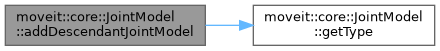Definition at line 267 of file joint_model.cpp.

 void moveit::core::JointModel::addMimicRequest ( const JointModel * joint )

Notify this joint that there is another joint that mimics it.

Definition at line 255 of file joint_model.cpp.

## ◆ computeTransform()

 virtual void moveit::core::JointModel::computeTransform ( const double * joint_values, Eigen::Isometry3d & transf ) const
pure virtual

Given the joint values for a joint, compute the corresponding transform. The computed transform is guaranteed to be a valid isometry.

Here is the caller graph for this function: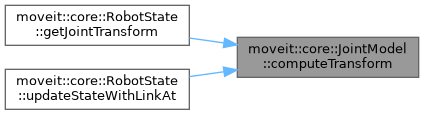## ◆ computeVariableBoundsMsg()

 void moveit::core::JointModel::computeVariableBoundsMsg ( )
protected

Definition at line 227 of file joint_model.cpp.

Here is the caller graph for this function: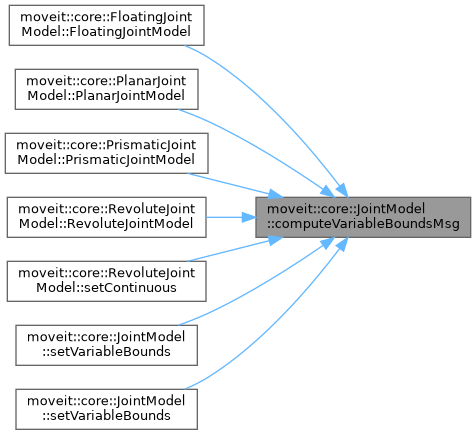## ◆ computeVariablePositions()

 virtual void moveit::core::JointModel::computeVariablePositions ( const Eigen::Isometry3d & transform, double * joint_values ) const
pure virtual

Given the transform generated by joint, compute the corresponding joint values. Make sure the passed transform is a valid isometry.

Here is the caller graph for this function: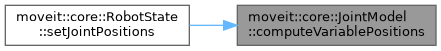## ◆ distance()

 virtual double moveit::core::JointModel::distance ( const double * value1, const double * value2 ) const
pure virtual

Compute the distance between two joint states of the same model (represented by the variable values)

Here is the caller graph for this function: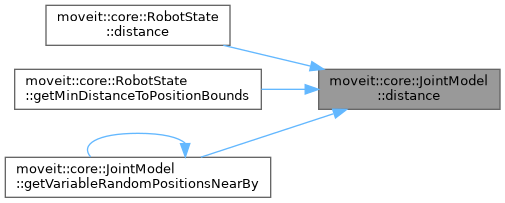## ◆ enforcePositionBounds() [1/2]

 bool moveit::core::JointModel::enforcePositionBounds ( double * values ) const
inline

Force the specified values to be inside bounds and normalized. Quaternions are normalized, continuous revolute joints are made between -Pi and Pi. Returns true if changes were made.

Definition at line 292 of file joint_model.h.

Here is the caller graph for this function: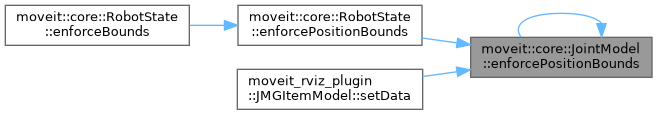## ◆ enforcePositionBounds() [2/2]

 virtual bool moveit::core::JointModel::enforcePositionBounds ( double * values, const Bounds & other_bounds ) const
pure virtual

Force the specified values to be inside bounds and normalized. Quaternions are normalized, continuous revolute joints are made between -Pi and Pi. Return true if changes were made.

## ◆ enforceVelocityBounds() [1/2]

 bool moveit::core::JointModel::enforceVelocityBounds ( double * values ) const
inline

Force the specified velocities to be within bounds. Return true if changes were made.

Definition at line 320 of file joint_model.h.

Here is the caller graph for this function: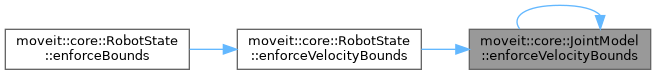## ◆ enforceVelocityBounds() [2/2]

 bool moveit::core::JointModel::enforceVelocityBounds ( double * values, const Bounds & other_bounds ) const
virtual

Force the specified velocities to be inside bounds. Return true if changes were made.

Definition at line 98 of file joint_model.cpp.

inline

Get the link that this joint connects to. There will always be such a link.

Definition at line 168 of file joint_model.h.

Here is the caller graph for this function:## ◆ getDescendantJointModels()

 const std::vector& moveit::core::JointModel::getDescendantJointModels ( ) const
inline

Get all the joint models that descend from this joint, in the kinematic tree.

Definition at line 428 of file joint_model.h.

 const std::vector& moveit::core::JointModel::getDescendantLinkModels ( ) const
inline

Get all the link models that descend from this joint, in the kinematic tree.

Definition at line 422 of file joint_model.h.

Here is the caller graph for this function:## ◆ getDistanceFactor()

 double moveit::core::JointModel::getDistanceFactor ( ) const
inline

Get the factor that should be applied to the value returned by distance() when that value is used in compound distances.

Definition at line 374 of file joint_model.h.

## ◆ getFirstVariableIndex()

 size_t moveit::core::JointModel::getFirstVariableIndex ( ) const
inline

Get the index of this joint's first variable within the full robot state.

Definition at line 216 of file joint_model.h.

Here is the caller graph for this function: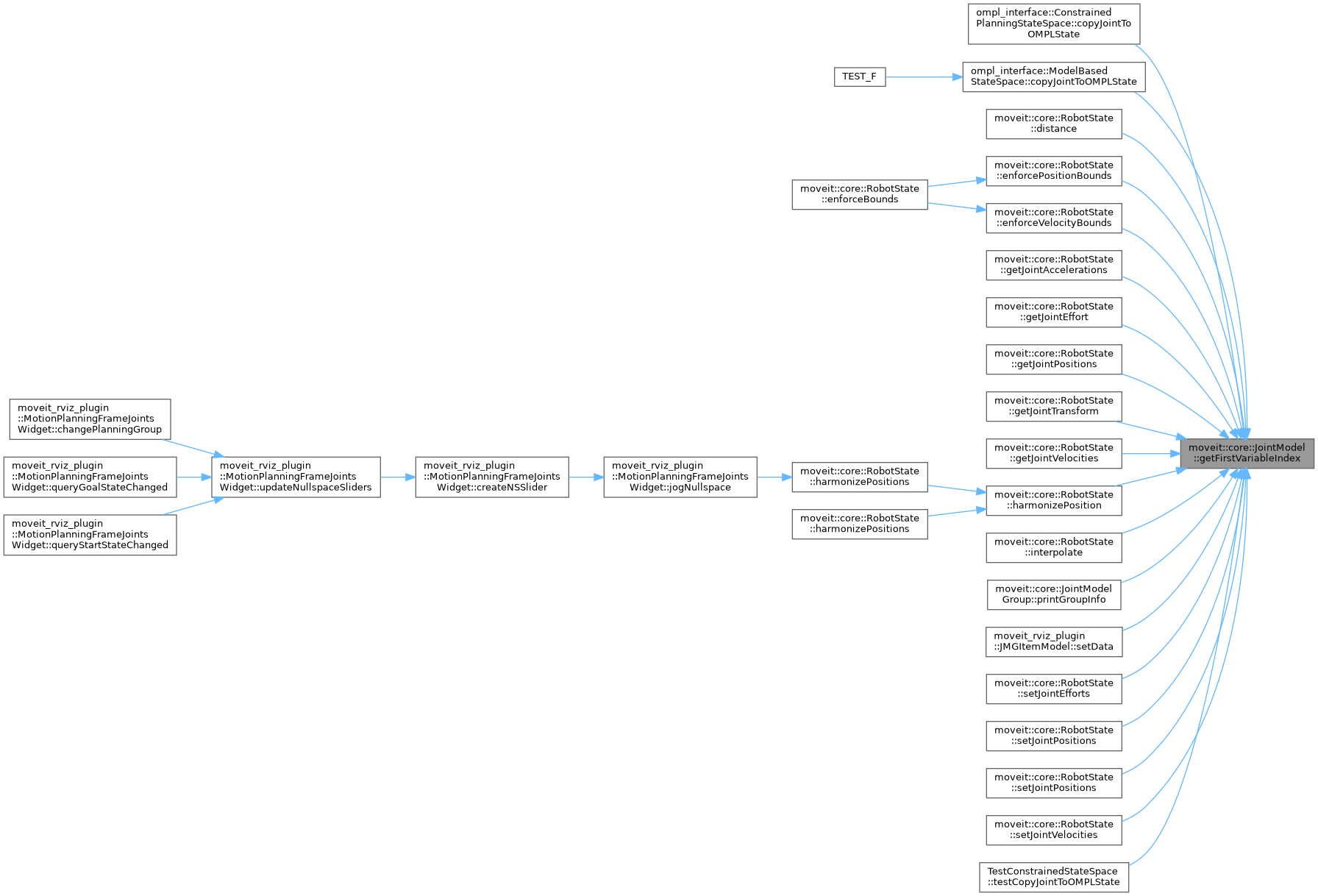## ◆ getJointIndex()

 size_t moveit::core::JointModel::getJointIndex ( ) const
inline

Get the index of this joint within the robot model.

Definition at line 222 of file joint_model.h.

Here is the caller graph for this function: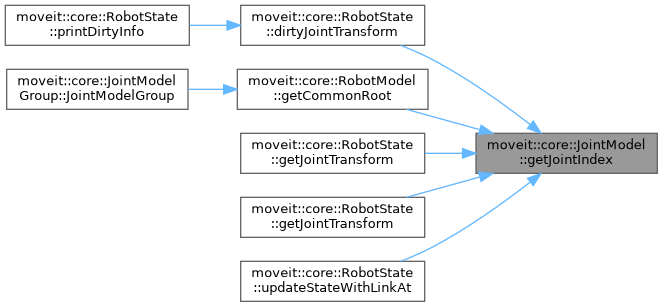## ◆ getLocalVariableIndex()

 size_t moveit::core::JointModel::getLocalVariableIndex ( const std::string & variable ) const

Get the index of the variable within this joint.

Definition at line 85 of file joint_model.cpp.

Here is the caller graph for this function: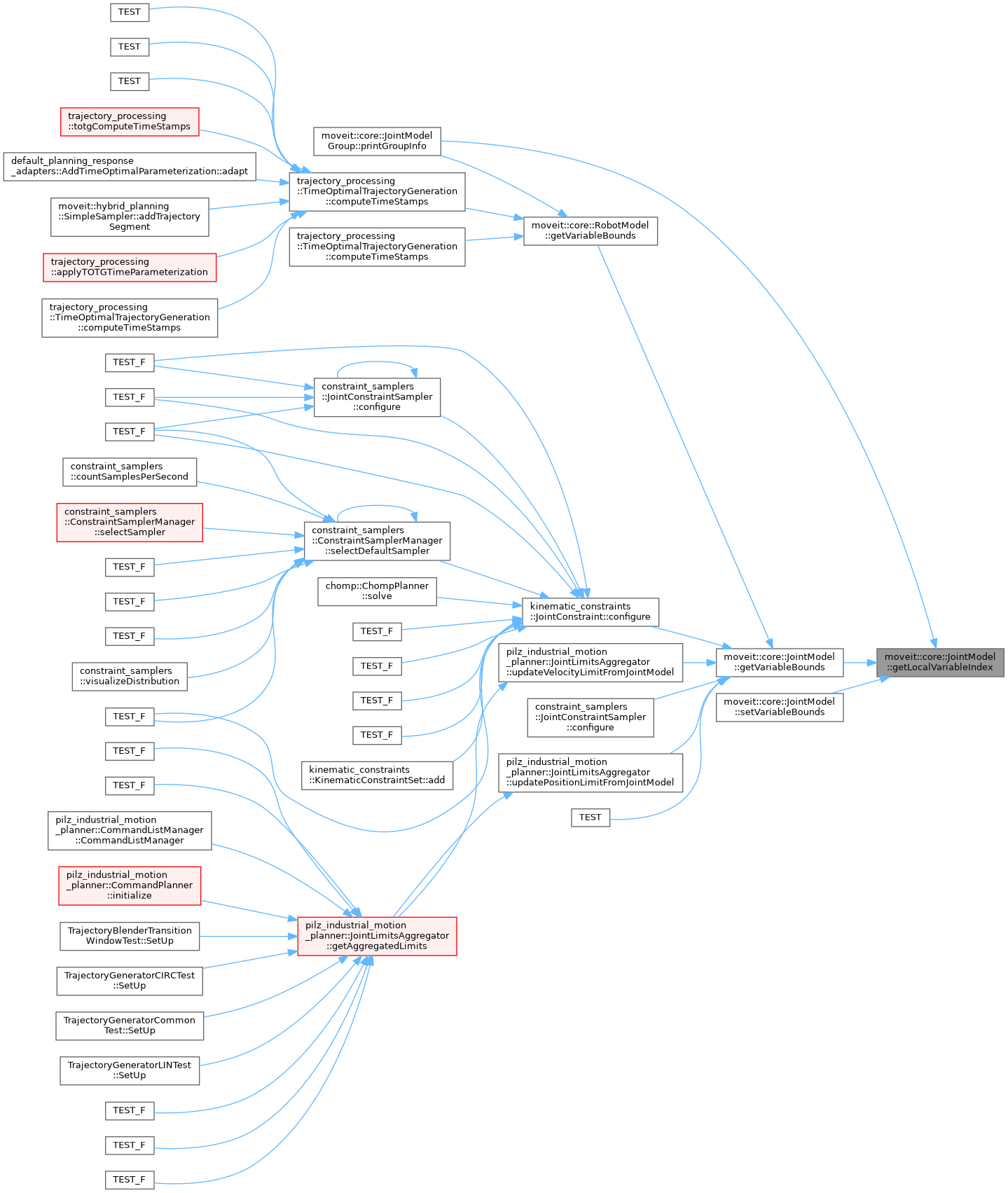## ◆ getLocalVariableNames()

 const std::vector& moveit::core::JointModel::getLocalVariableNames ( ) const
inline

Get the local names of the variable that make up the joint (suffixes that are attached to joint names to construct the variable names). For single DOF joints, this will be empty.

Definition at line 198 of file joint_model.h.

Here is the caller graph for this function:## ◆ getMaximumExtent() [1/2]

 double moveit::core::JointModel::getMaximumExtent ( ) const
inline

Definition at line 459 of file joint_model.h.

## ◆ getMaximumExtent() [2/2]

 virtual double moveit::core::JointModel::getMaximumExtent ( const Bounds & other_bounds ) const
pure virtual

Get the extent of the state space (the maximum value distance() can ever report)

## ◆ getMimic()

 const JointModel* moveit::core::JointModel::getMimic ( ) const
inline

Get the joint this one is mimicking.

Definition at line 390 of file joint_model.h.

Here is the caller graph for this function:## ◆ getMimicFactor()

 double moveit::core::JointModel::getMimicFactor ( ) const
inline

If mimicking a joint, this is the multiplicative factor for that joint's value.

Definition at line 402 of file joint_model.h.

Here is the caller graph for this function: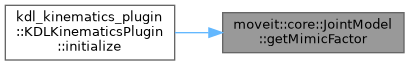## ◆ getMimicOffset()

 double moveit::core::JointModel::getMimicOffset ( ) const
inline

If mimicking a joint, this is the offset added to that joint's value.

Definition at line 396 of file joint_model.h.

Here is the caller graph for this function: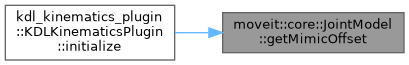## ◆ getMimicRequests()

 const std::vector& moveit::core::JointModel::getMimicRequests ( ) const
inline

The joint models whose values would be modified if the value of this joint changed.

Definition at line 411 of file joint_model.h.

## ◆ getName()

 const std::string& moveit::core::JointModel::getName ( ) const
inline

Get the name of the joint.

Definition at line 145 of file joint_model.h.

Here is the caller graph for this function:## ◆ getNonFixedDescendantJointModels()

 const std::vector& moveit::core::JointModel::getNonFixedDescendantJointModels ( ) const
inline

Get all the non-fixed joint models that descend from this joint, in the kinematic tree.

Definition at line 434 of file joint_model.h.

inline

Get the link that this joint connects to. The robot is assumed to start with a joint, so the root joint will return nullptr here.

Definition at line 162 of file joint_model.h.

Here is the caller graph for this function:## ◆ getStateSpaceDimension()

 virtual unsigned int moveit::core::JointModel::getStateSpaceDimension ( ) const
pure virtual

Get the dimension of the state space that corresponds to this joint.

## ◆ getType()

 JointType moveit::core::JointModel::getType ( ) const
inline

Get the type of joint.

Definition at line 151 of file joint_model.h.

Here is the caller graph for this function: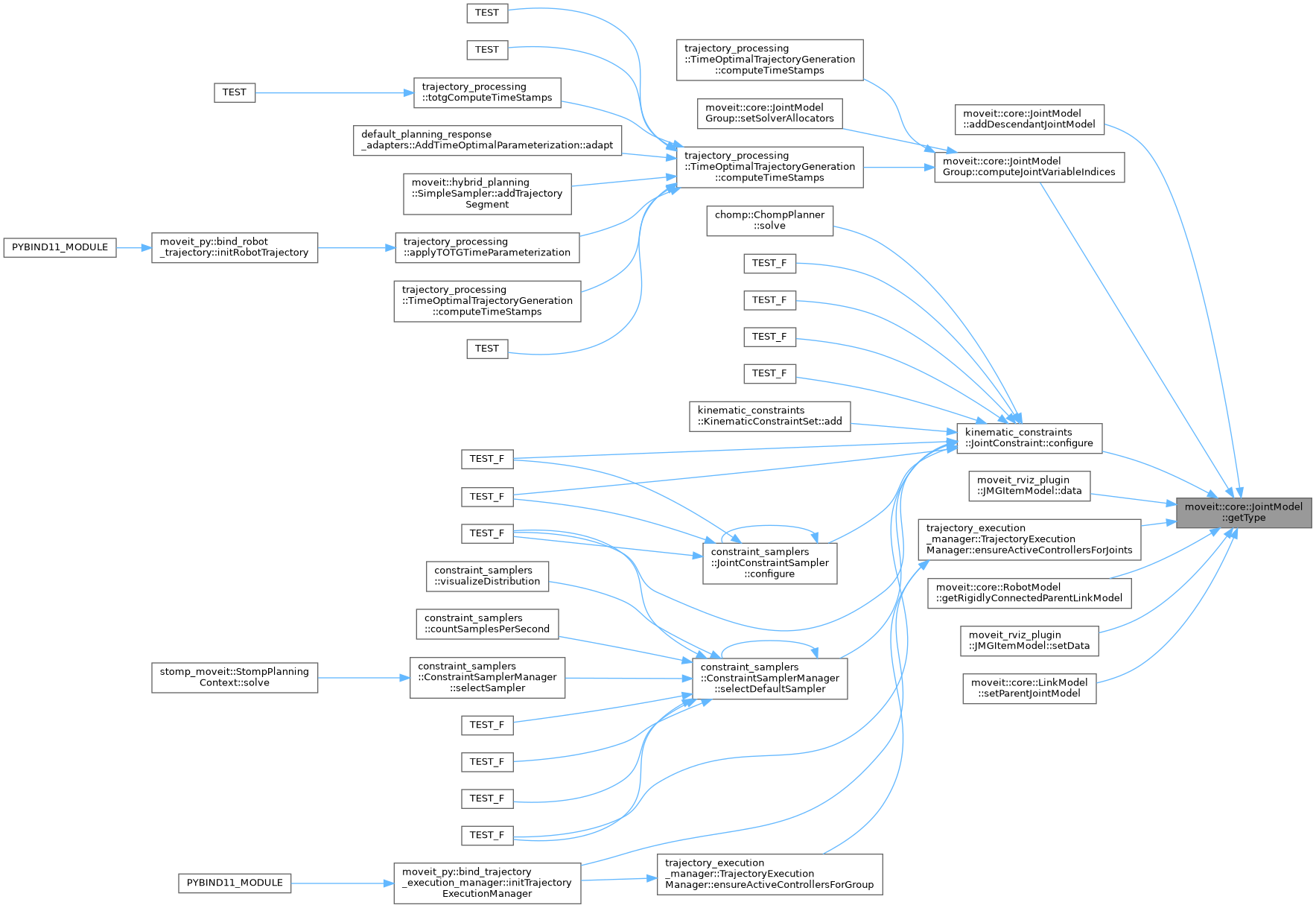## ◆ getTypeName()

 std::string moveit::core::JointModel::getTypeName ( ) const

Get the type of joint as a string.

Definition at line 64 of file joint_model.cpp.

Here is the caller graph for this function: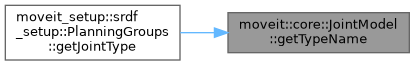## ◆ getVariableBounds() [1/2]

 const Bounds& moveit::core::JointModel::getVariableBounds ( ) const
inline

Get the variable bounds for this joint, in the same order as the names returned by getVariableNames()

Definition at line 350 of file joint_model.h.

## ◆ getVariableBounds() [2/2]

 const VariableBounds & moveit::core::JointModel::getVariableBounds ( const std::string & variable ) const

Get the bounds for a variable. Throw an exception if the variable was not found.

Definition at line 177 of file joint_model.cpp.

Here is the call graph for this function: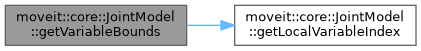Here is the caller graph for this function: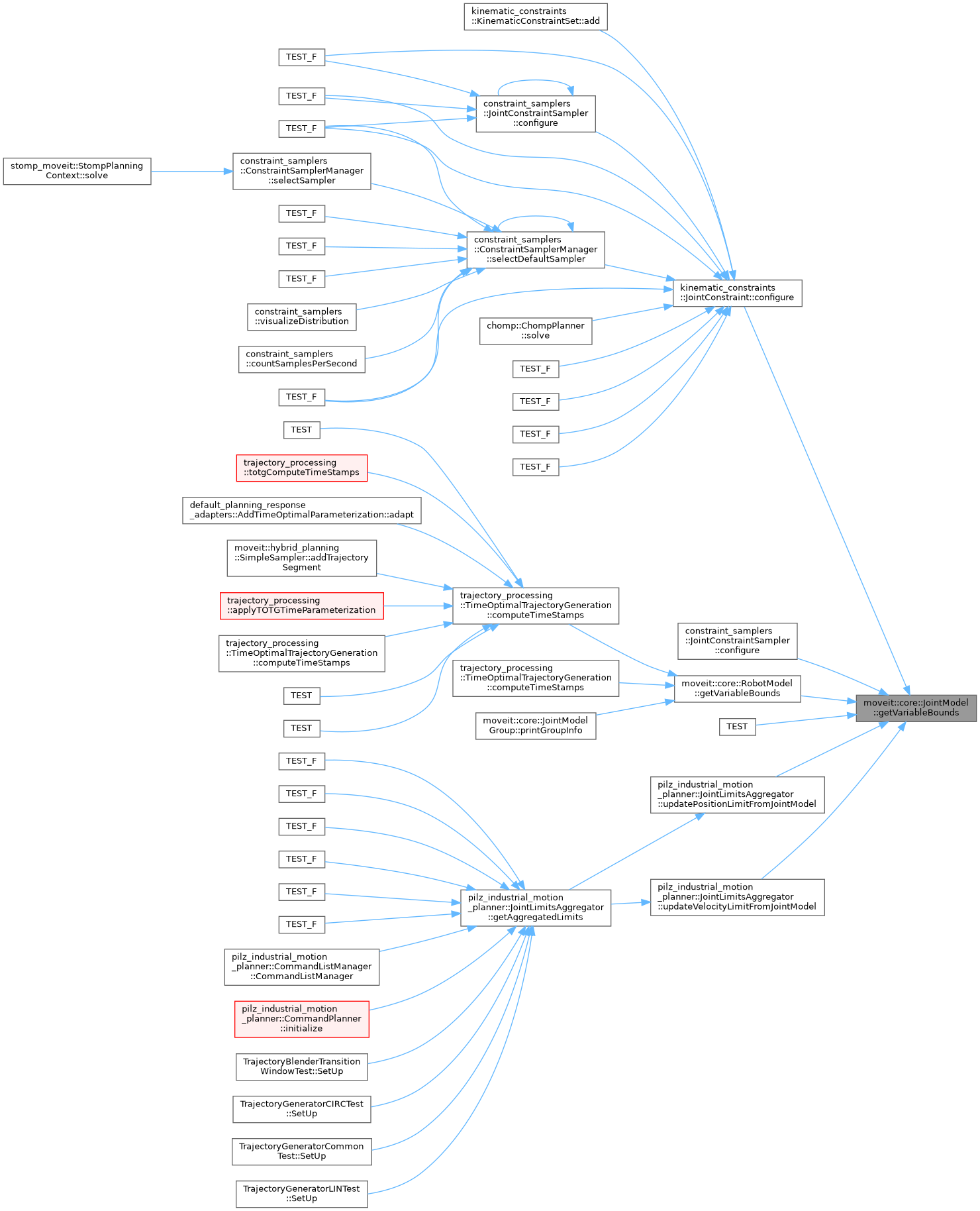## ◆ getVariableBoundsMsg()

 const std::vector& moveit::core::JointModel::getVariableBoundsMsg ( ) const
inline

Get the joint limits known to this model, as a message.

Definition at line 362 of file joint_model.h.

Here is the caller graph for this function:## ◆ getVariableCount()

 std::size_t moveit::core::JointModel::getVariableCount ( ) const
inline

Get the number of variables that describe this joint.

Definition at line 210 of file joint_model.h.

Here is the caller graph for this function: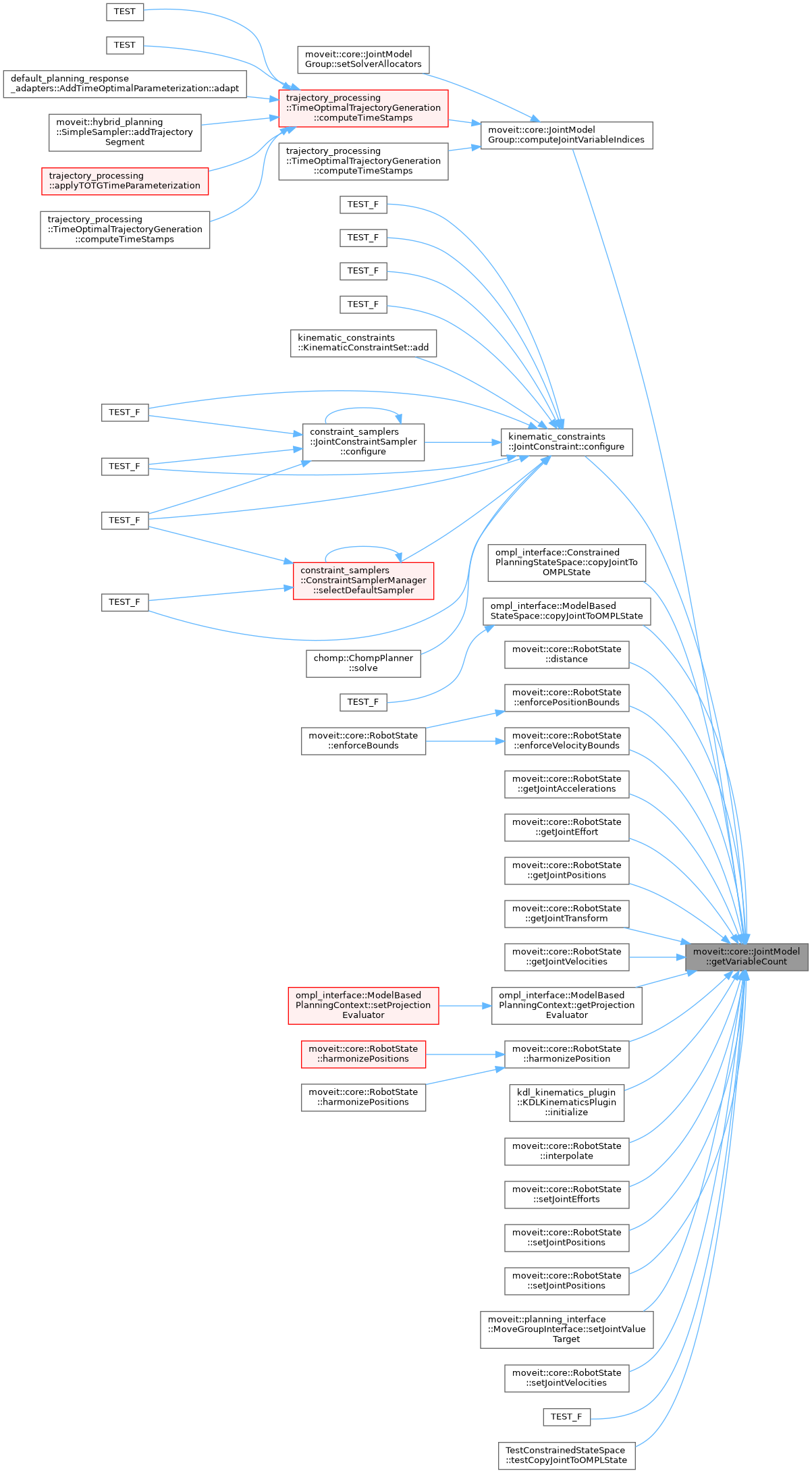## ◆ getVariableDefaultPositions() [1/2]

 void moveit::core::JointModel::getVariableDefaultPositions ( double * values ) const
inline

Provide a default value for the joint given the default joint variable bounds (maintained internally). Most joints will use the default implementation provided in this base class, but the quaternion for example needs a different implementation. Enough memory is assumed to be allocated.

Definition at line 238 of file joint_model.h.

## ◆ getVariableDefaultPositions() [2/2]

 virtual void moveit::core::JointModel::getVariableDefaultPositions ( double * values, const Bounds & other_bounds ) const
pure virtual

Provide a default value for the joint given the joint variable bounds. Most joints will use the default implementation provided in this base class, but the quaternion for example needs a different implementation. Enough memory is assumed to be allocated.

## ◆ getVariableNames()

 const std::vector& moveit::core::JointModel::getVariableNames ( ) const
inline

Get the names of the variables that make up this joint, in the order they appear in corresponding states. For single DOF joints, this will be just the joint name. For multi-DOF joints these will be the joint name followed by "/", followed by the local names of the variables.

Definition at line 190 of file joint_model.h.

## ◆ getVariableRandomPositions() [1/2]

 void moveit::core::JointModel::getVariableRandomPositions ( random_numbers::RandomNumberGenerator & rng, double * values ) const
inline

Provide random values for the joint variables (within default bounds). Enough memory is assumed to be allocated.

Definition at line 250 of file joint_model.h.

## ◆ getVariableRandomPositions() [2/2]

 virtual void moveit::core::JointModel::getVariableRandomPositions ( random_numbers::RandomNumberGenerator & rng, double * values, const Bounds & other_bounds ) const
pure virtual

Provide random values for the joint variables (within specified bounds). Enough memory is assumed to be allocated.

## ◆ getVariableRandomPositionsNearBy() [1/2]

 virtual void moveit::core::JointModel::getVariableRandomPositionsNearBy ( random_numbers::RandomNumberGenerator & rng, double * values, const Bounds & other_bounds, const double * near, const double distance ) const
pure virtual

Provide random values for the joint variables (within specified bounds). Enough memory is assumed to be allocated.

## ◆ getVariableRandomPositionsNearBy() [2/2]

 void moveit::core::JointModel::getVariableRandomPositionsNearBy ( random_numbers::RandomNumberGenerator & rng, double * values, const double * near, const double distance ) const
inline

Provide random values for the joint variables (within default bounds). Enough memory is assumed to be allocated.

Definition at line 262 of file joint_model.h.

Here is the call graph for this function: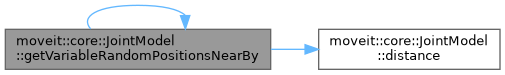## ◆ harmonizePosition() [1/2]

 bool moveit::core::JointModel::harmonizePosition ( double * values ) const
inline

Definition at line 305 of file joint_model.h.

Here is the call graph for this function: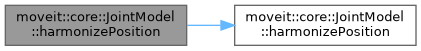## ◆ harmonizePosition() [2/2]

 bool moveit::core::JointModel::harmonizePosition ( double * values, const Bounds & other_bounds ) const
virtual

Harmonize position of revolute joints, adding/subtracting multiples of 2*Pi to bring them back into bounds. Return true if changes were made.

Reimplemented in moveit::core::RevoluteJointModel.

Definition at line 93 of file joint_model.cpp.

Here is the caller graph for this function: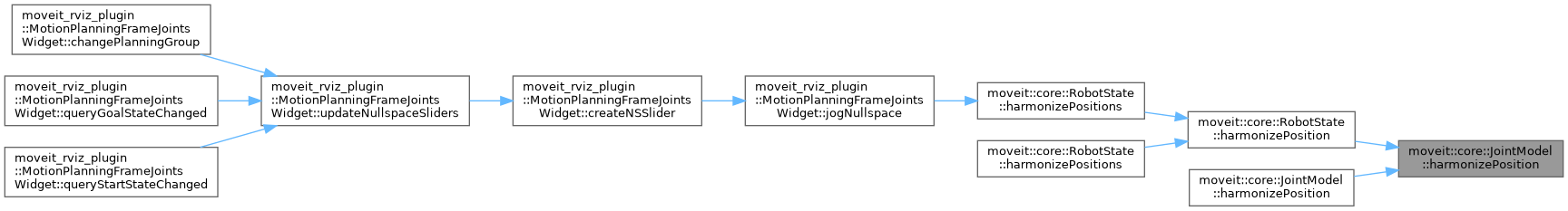## ◆ hasVariable()

 bool moveit::core::JointModel::hasVariable ( const std::string & variable ) const
inline

Check if a particular variable is known to this joint.

Definition at line 204 of file joint_model.h.

## ◆ interpolate()

 virtual void moveit::core::JointModel::interpolate ( const double * from, const double * to, const double t, double * state ) const
pure virtual

Computes the state that lies at time t in [0, 1] on the segment that connects from state to to state. The memory location of state is not required to be different from the memory of either from or to.

Here is the caller graph for this function: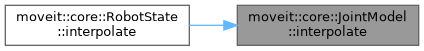## ◆ isPassive()

 bool moveit::core::JointModel::isPassive ( ) const
inline

Check if this joint is passive.

Definition at line 440 of file joint_model.h.

Here is the caller graph for this function: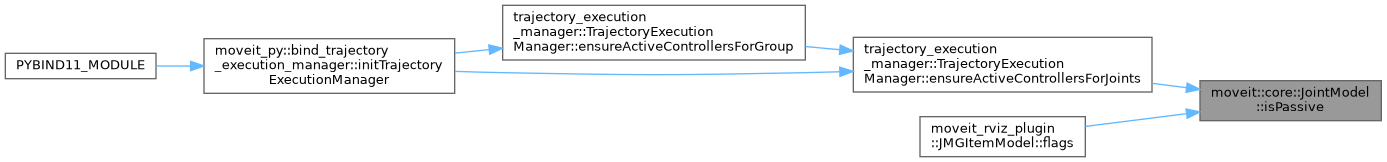## ◆ satisfiesAccelerationBounds() [1/2]

 bool moveit::core::JointModel::satisfiesAccelerationBounds ( const double * values, const Bounds & other_bounds, double margin ) const
virtual

Check if the set of accelerations for the variables of this joint are within bounds, up to some margin.

Definition at line 137 of file joint_model.cpp.

## ◆ satisfiesAccelerationBounds() [2/2]

 bool moveit::core::JointModel::satisfiesAccelerationBounds ( const double * values, double margin = `0.0` ) const
inline

Check if the set of accelerations for the variables of this joint are within bounds.

Definition at line 329 of file joint_model.h.

## ◆ satisfiesJerkBounds() [1/2]

 bool moveit::core::JointModel::satisfiesJerkBounds ( const double * values, const Bounds & other_bounds, double margin ) const
virtual

Check if the set of jerks for the variables of this joint are within bounds, up to some margin.

Definition at line 157 of file joint_model.cpp.

## ◆ satisfiesJerkBounds() [2/2]

 bool moveit::core::JointModel::satisfiesJerkBounds ( const double * values, double margin = `0.0` ) const
inline

Check if the set of jerks for the variables of this joint are within bounds.

Definition at line 338 of file joint_model.h.

## ◆ satisfiesPositionBounds() [1/2]

 virtual bool moveit::core::JointModel::satisfiesPositionBounds ( const double * values, const Bounds & other_bounds, double margin ) const
pure virtual

Check if the set of position values for the variables of this joint are within bounds, up to some margin.

## ◆ satisfiesPositionBounds() [2/2]

 bool moveit::core::JointModel::satisfiesPositionBounds ( const double * values, double margin = `0.0` ) const
inline

Check if the set of values for the variables of this joint are within bounds.

Definition at line 280 of file joint_model.h.

Here is the caller graph for this function: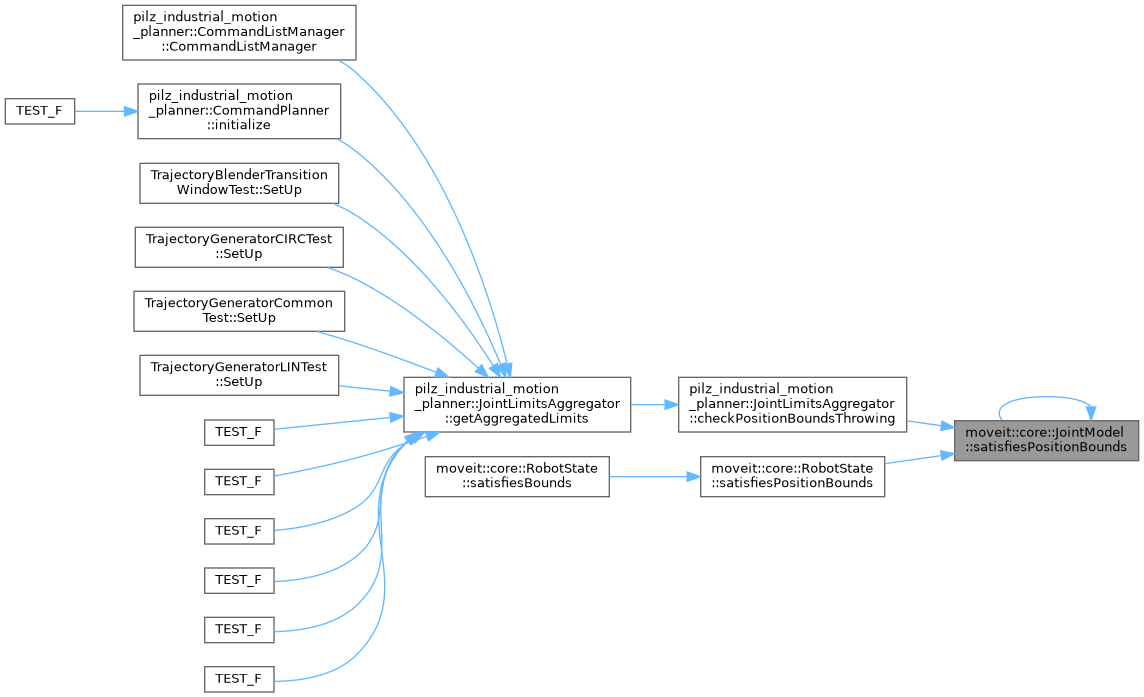## ◆ satisfiesVelocityBounds() [1/2]

 bool moveit::core::JointModel::satisfiesVelocityBounds ( const double * values, const Bounds & other_bounds, double margin ) const
virtual

Check if the set of velocities for the variables of this joint are within bounds, up to some margin.

Definition at line 117 of file joint_model.cpp.

## ◆ satisfiesVelocityBounds() [2/2]

 bool moveit::core::JointModel::satisfiesVelocityBounds ( const double * values, double margin = `0.0` ) const
inline

Check if the set of velocities for the variables of this joint are within bounds.

Definition at line 311 of file joint_model.h.

Here is the caller graph for this function: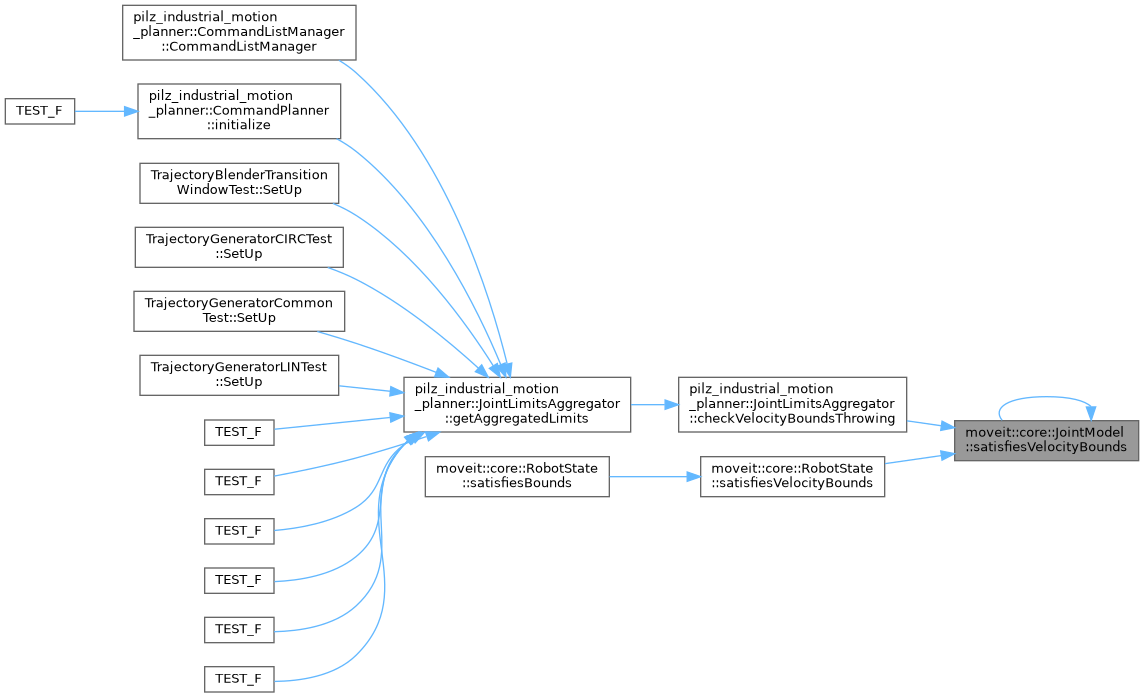inline

Definition at line 178 of file joint_model.h.

## ◆ setDistanceFactor()

 void moveit::core::JointModel::setDistanceFactor ( double factor )
inline

Set the factor that should be applied to the value returned by distance() when that value is used in compound distances.

Definition at line 381 of file joint_model.h.

## ◆ setMimic()

 void moveit::core::JointModel::setMimic ( const JointModel * mimic, double factor, double offset )

Mark this joint as mimicking mimic using factor and offset.

Definition at line 248 of file joint_model.cpp.

inline

Definition at line 173 of file joint_model.h.

## ◆ setPassive()

 void moveit::core::JointModel::setPassive ( bool flag )
inline

Definition at line 445 of file joint_model.h.

## ◆ setVariableBounds() [1/2]

 void moveit::core::JointModel::setVariableBounds ( const std::string & variable, const VariableBounds & bounds )

Set the lower and upper bounds for a variable. Throw an exception if the variable was not found.

Definition at line 182 of file joint_model.cpp.

Here is the call graph for this function: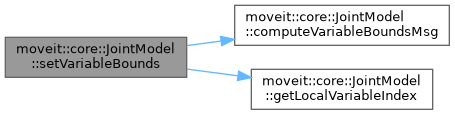## ◆ setVariableBounds() [2/2]

 void moveit::core::JointModel::setVariableBounds ( const std::vector< moveit_msgs::msg::JointLimits > & jlim )

Override joint limits loaded from URDF. Unknown variables are ignored.

Definition at line 188 of file joint_model.cpp.

Here is the call graph for this function: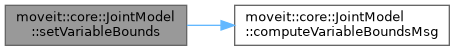## Member Data Documentation

protected

Definition at line 512 of file joint_model.h.

## ◆ descendant_joint_models_

 std::vector moveit::core::JointModel::descendant_joint_models_
protected

Pointers to all the joints that follow this one in the kinematic tree (including mimic joints)

Definition at line 530 of file joint_model.h.

protected

Pointers to all the links that will be moved if this joint changes value.

Definition at line 527 of file joint_model.h.

## ◆ distance_factor_

 double moveit::core::JointModel::distance_factor_
protected

The factor applied to the distance between two joint states.

Definition at line 540 of file joint_model.h.

## ◆ local_variable_names_

 std::vector moveit::core::JointModel::local_variable_names_
protected

The local names to use for the variables that make up this joint.

Definition at line 494 of file joint_model.h.

## ◆ mimic_

 const JointModel* moveit::core::JointModel::mimic_
protected

The joint this one mimics (nullptr for joints that do not mimic)

Definition at line 515 of file joint_model.h.

## ◆ mimic_factor_

 double moveit::core::JointModel::mimic_factor_
protected

The offset to the mimic joint.

Definition at line 518 of file joint_model.h.

## ◆ mimic_offset_

 double moveit::core::JointModel::mimic_offset_
protected

The multiplier to the mimic joint.

Definition at line 521 of file joint_model.h.

## ◆ mimic_requests_

 std::vector moveit::core::JointModel::mimic_requests_
protected

The set of joints that should get a value copied to them when this joint changes.

Definition at line 524 of file joint_model.h.

## ◆ non_fixed_descendant_joint_models_

 std::vector moveit::core::JointModel::non_fixed_descendant_joint_models_
protected

Pointers to all the joints that follow this one in the kinematic tree, including mimic joints, but excluding fixed joints.

Definition at line 534 of file joint_model.h.

protected

Definition at line 509 of file joint_model.h.

## ◆ passive_

 bool moveit::core::JointModel::passive_
protected

Specify whether this joint is marked as passive in the SRDF.

Definition at line 537 of file joint_model.h.

## ◆ type_

 JointType moveit::core::JointModel::type_
protected

The type of joint.

Definition at line 491 of file joint_model.h.

## ◆ variable_bounds_

 Bounds moveit::core::JointModel::variable_bounds_
protected

The bounds for each variable (low, high) in the same order as variable_names_.

Definition at line 500 of file joint_model.h.

## ◆ variable_bounds_msg_

 std::vector moveit::core::JointModel::variable_bounds_msg_
protected

Definition at line 502 of file joint_model.h.

## ◆ variable_index_map_

 VariableIndexMap moveit::core::JointModel::variable_index_map_
protected

Map from variable names to the corresponding index in variable_names_ (indexing makes sense within the JointModel only)

Definition at line 506 of file joint_model.h.

## ◆ variable_names_

 std::vector moveit::core::JointModel::variable_names_
protected

The full names to use for the variables that make up this joint.

Definition at line 497 of file joint_model.h.

The documentation for this class was generated from the following files: